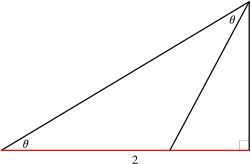#### You may also likeThis comes in two parts, with the first being less fiendish than the second. Itâ€™s great for practising both quadratics and laws of indices, and you can get a lot from making sure that you find all the solutions. For a real challenge (requiring a bit more knowledge), you could consider finding the complex solutions.### Discriminating

You're invited to decide whether statements about the number of solutions of a quadratic equation are always, sometimes or never true.This will encourage you to think about whether all quadratics can be factorised and to develop a better understanding of the effect that changing the coefficients has on the factorised form.

# $t$ for tan

##### Age 16 to 18Challenge LevelThis resource is from Underground Mathematics.

If we write $t = \tan \theta$, then the following equations are true.

\begin{align*}
\tan(2\theta) &= \frac{2t}{1-t^2}, \\
\sin(2\theta) &= \frac{2t}{1+t^2}, \\
\cos(2\theta) &= \frac{1-t^2}{1+t^2}.
\end{align*}

Can you use this diagram to obtain these formulae?For what range of values of $\theta$ does this argument work?

This is an Underground Mathematics resource.

Underground Mathematics is hosted by Cambridge Mathematics. The project was originally funded by a grant from the UK Department for Education to provide free web-based resources that support the teaching and learning of post-16 mathematics.

Visit the site at undergroundmathematics.org to find more resources, which also offer suggestions, solutions and teacher notes to help with their use in the classroom.